# G.1 Matrix Truncation

By defining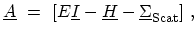(G.1)

the equation (4.11) (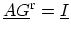) can be written as,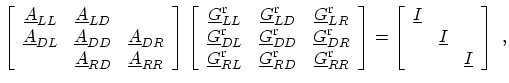(G.2)

where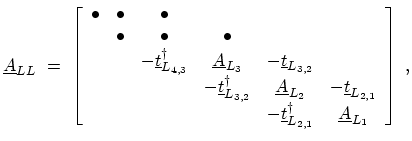(G.3)

corresponds to the left semi-infinite contact,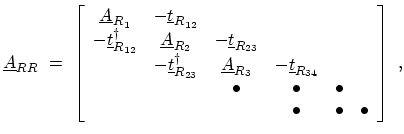(G.4)

corresponds to the right semi-infinite contact, and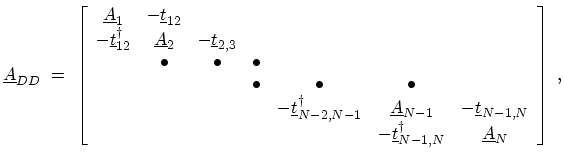(G.5)

corresponds to the device region.

The coupling between the left and right contacts and device are respectively given by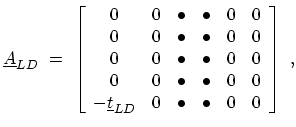(G.6)

and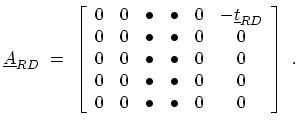(G.7)

It should be noted that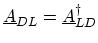,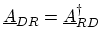, and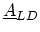and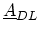(, and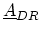) are sparse matrices. Their only non-zero entry represents the coupling of the left (right) contact and device. From (G.2), one obtains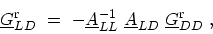(G.8)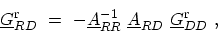(G.9)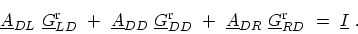(G.10)

Substituting (G.8) and (G.9) in (G.10), one obtains a matrix equation with a dimension corresponding to the total number of grid points in device layers,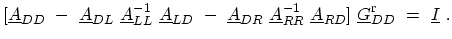(G.11)

The second and third terms of (G.11) are self-energies due to coupling of the device region to left and right contacts, respectively.

The GREEN's functions of the isolated semi-infinite contacts are defined as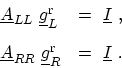(G.12)

The surface GREEN's function of the left and right contacts are the GREEN's function elements corresponding to the first edge layer of the respective contact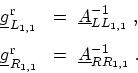(G.13)

M. Pourfath: Numerical Study of Quantum Transport in Carbon Nanotube-Based Transistors